## Maple2021 and CUDA...Examples

 >>>>(1.1)
 >(1.2)
 >(1.3)
 >(1.4)
 >(1.5)
 >(1.6)
 >(1.7)
 >(1.8)Hello all :-)

I just upgraded to 2021 and ran the example help page for CUDA.

From the help we can see that the ratio is 11.

With Maple 2020 I had a ratio of 7.24

With Maple 2021 I have a ratio of around 4. (see the mw attached).

I wonder with which version the help page was made but on my machines the ratio diminishes from version to version

Now it's Windows 10 Pro 20H2, the amazing AMD Ryzen Threadripper (64 cores) and 256 Go RAM, the graphic card is a NVIDIA RTX 3090 with...10496 CUDA cores.

So what can't I get a better ratio with/without CUDA?

Regards.

## Can we create more graphs with this function Sequ...

I get the degree sequence of a graph, I want to create more graphs, but maple seems to only get the only graph.

Is there a good way to get more, a foolish dream, can we get all the graphs that satisfy this degree sequence condition?

with(GraphTheory):
L:=[7\$1..22,6]:
IsGraphicSequence(L):
G := SequenceGraph(L):
DrawGraph(%)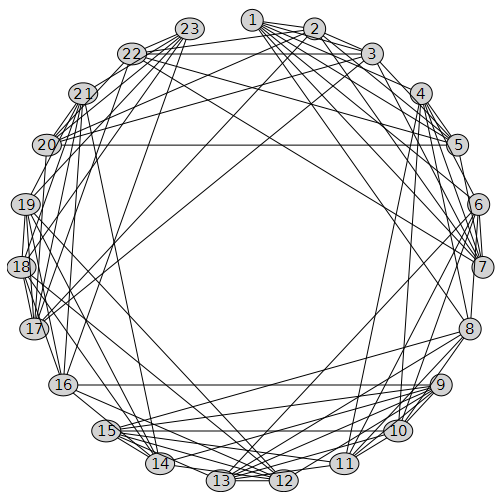## Problem with animation toolbar in Maple 2021...

Anyone have a problem with non-appearance of the animation toolbar in Maple 2021????

Whilst coming up with a response for the problem here

https://www.mapleprimes.com/questions/231862-Have-You-Ever-Heard-Of-Vector-Asterisks-

I found an issue with the non-appearance of the animation toolbar in Maple 2021. This is rather difficult to illustrate without the use of screenshots, for which I apologise.

Normally(?) I would just select a plot and the animation toolbar appears "as if by magic"

First screen shot is using Maple 2020, The blue highlighting rectangle around the plot was visible when I initiated the snip, but disappeared when the snipping tool activated. However this shows that the animation toolbar is available (and works)I do exactly the same thing in  Maple 2021 and I can't make the animation toolbar appear - see below. Aagain the plot wa highlighted when I initiated the snip but the highlighting disappeared when the snipping tool activated. Now there is no sign of the animation toolbarIt is still possible to do very basic animation in Maple 2021 by clicking on the plot and using the context menu - but this is very basic

The code used in the above plots is given supplied below

```  restart;
kernelopts(version);
plots:-display
( [ seq
( plot
( Vector([1, 3, 4, 6]),
Vector([8, 6, 2, 5]),
style = point,
symbol = j,
symbolsize = 40,
color = blue
),
j in [ asterisk, box, circle, cross, diagonalcross,
diamond, point, solidbox, solidcircle, soliddiamond
]
)
],
insequence = true
);
```

Is it just me?

## Does table have a predefined function to get indic...

Consider an easy example of a table.

`myTable:=table(["a"=1,"b"=-1,"c"=1]);`

We can give an index to it and get its corresponding entry if exists.

`myTable["b"];`

We can also get the set of all indices, or the set of all entries. But what about receiving the index or set of indices with a specific entry. for example asking what indices have the entry `1`?

Of course I can define a search procedure myself, but I thought there might be an efficient way which is already implemented as a function/method on tables.

## pdsolve exception (in GAMMA) numeric exception: di...

This is new exception generated by Maple pdsolve in 2021. Different from the last post I gave on pdsolve. So I thought it will be better to keep them separate since the causes are different.

```interface(version)
restart;
pde :=  diff(w(x,y,z),x)+(a1*x^n1*y+b1*x^m1)*diff(w(x,y,z),y)+(a2*x^n2*y+b2*x^m1)*diff(w(x,y,z),z)= 0;
pdsolve(pde,w(x,y,z));

#another example

restart;
local gamma:
pde := diff(w(x,y,z),x)+(a1*x^n1*y+ b1*x^m1)*diff(w(x,y,z),y)+(a2*x^n2*y+b2*x^m2)*diff(w(x,y,z),z)=c2*x^k2*y+c1*x^k1*z;
pdsolve(pde,w(x,y,z));

```

Error, (in GAMMA) numeric exception: division by zero

The same PDE works in 2020.2. The answer it gives is large so will not show it all below.

Screen shots

Maple 2021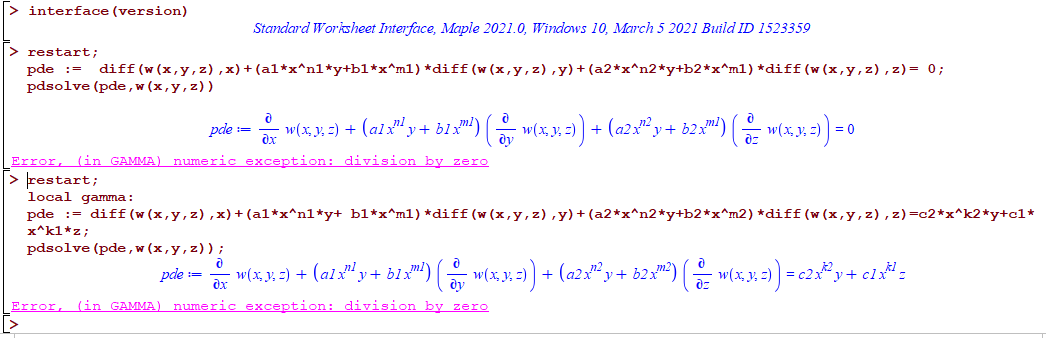Maple 2020.2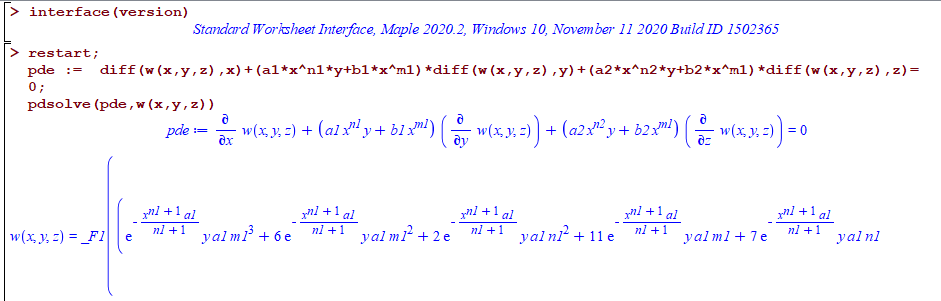All on windows 10.

Do other see the same error? What causes it?

## seq - numelems and declaring a local variable in ...## pdsolve exception int/gbinthm/structure INVALID s...

I noticed number of pde's now fail in Maple 2021 with the error

int/gbinthm/structure INVALID subscript selector

but they do not fail in Maple 2020.2.

Here are few   examples

```restart;
pde :=a*x^n*diff(w(x,y),x) + n*x^m*y*diff(w(x,y),y) =s*x^p*y^q+d;
pdsolve(pde,w(x,y));

restart;
pde :=a*x^n*diff(w(x,y),x) + n*x^m*y*diff(w(x,y),y)=c*x^k*y^s+d;
pdsolve(pde,w(x,y))

restart;
pde :=a*x^n*diff(w(x,y),x)+b*x^m*y*diff(w(x,y),y) =  (c*x^k*y^s + d)*w(x,y);
pdsolve(pde,w(x,y))

restart;
pde :=  a*diff(w(x,y),x)+ y*diff(w(x,y),y) = b*w(x,y)+ c*x^n*y^m;
pdsolve(pde,w(x,y))

#etc..```

Error, (in int/gbinthm/structure) invalid subscript selector

While in Maple 2020.2 they all work. Screen shot

Maple 2021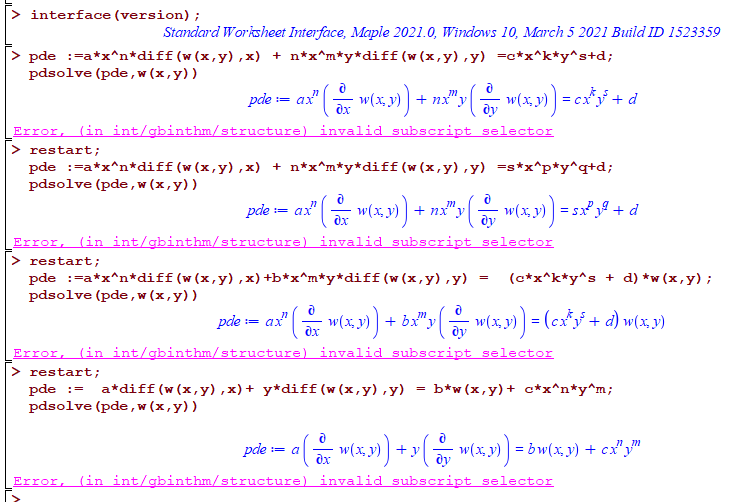Maple 2020.2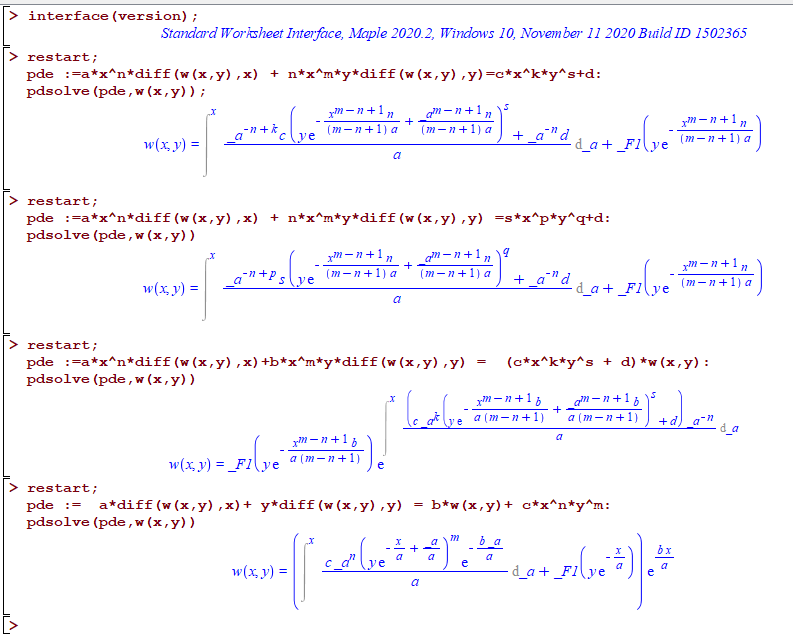Any idea why this happens? Do others see the same error?

## Maple 2021 / Problem while saving the workbook...

I've experienced problems a couple of times when trying to save a workbook in Maple 2021.

At first I thought it was due to a unstable VPN connection, but this also happens on a local drive.## why the new documentation PDF's for Maple 2021 are...

This is just an obervation. May be due to me missing some clarification.

My understanding is that in Maple 2021, one can now export a complete Maple worksheet/document to Latex successfuly.

According to https://www.maplesoft.com/products/maple/new_features/  it says under "Latex export"

Export is available for individual expressions or the entire document, though the latex command

So I would have expected that now all the online PDF documents for the new features in Maple 2021 to be generated from Latex for better formatting.

But when going over number of documents, they do not appear to have converted to Latex before generating the PDF.

Even the PDF document about the new latex command does not appear to have been generated from Latex. I can quickly recognize a PDF that was generated from Latex, and these PDF files are clearly not.

Looking at sample PDF from Maple website, it says the generator is "iText" and not latex compiler.

It looks like these documents were just a direct export of the Maple worksheet to PDF, instead of being exporting to Latex first, and then compiled to PDF.

Am I missing something? Why not take advantage of the Maple Latex export, and convert all the documents to Latex first and then generate the PDF from Latex? This would make them look much better in terms of formatting and math rendering.

Here are some examples

Why did not Maple documentation take advantage of the new export command of Maple's latex command? This would also have been a good way and an opportunity to show case the effectiveness of the new latex export command.

May be it was not done due to time constraint before the release date of maple 2021? Or are there other technical reasons?

## Bug in Integration of square of Jacobi sine functi...

Dear all,

I believe there is a bug in integration of square of Jacobi sine function.

Here is what I did:

u := int(JacobiSN(x, k)^2, x);

The result I got is
x - EllipticE(JacobiSN(x, k), k)
u := --------------------------------
k^2
However, according to  Handbook of elliptic integrals for engineers and scientists by Byrd and Friedman Eq (310.02), it is supposed to be

x - EllipticE(JacobiAM(x, k), k)
u := --------------------------------
k^2

Note that I tried also to integrate first and third power of JacobiSN a the results are in agreement with the book.

## Some bug with Physics and int in Maple 2021...

Hi there.

I found some strange behavior of int function in document with using of Physics package in Maple 2021 in comparison with Maple 2020:

kerr.mw

Thank you.

## How do I Int(pde) in Maple?...

Int(UxUxy+UxxUy, x)=UxUy

How can I do this?

## Polynomials: How do I determine the linear factors...

Show that x-2 is a factor of p(x)=x^3+3x^2-4x-12

I)determine all the linear factors of p(x)

ii)what are the zeros of y=p(x)

iii)sketch function y=p(x)

## issue using simplify on result of integration in ...

In Maple 2021

```restart;
B:=sqrt( (-4*u^(1/3)+1)*u^(4/3));
A:=1/(-12*u+3*u^(2/3)-3*B);
res:=int(A,u);
simplify(res)
```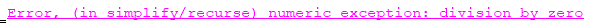This is one of the integrals, that in Maple 2020 did not handle. In Maple 2020, it gives this

```restart;
B:=sqrt( (-4*u^(1/3)+1)*u^(4/3));
A:=1/( -12*u+ 3*u^(2/3)-3*B);
res:=int(A,u);
```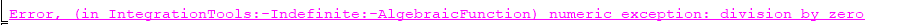The difference is that in Maple 2021 int is able to solve this (but gives very long output which I will not post here). The problem is now simplify() gives an error on the result. I was trying to see if possible to obtain the same small result as Mathematica's after simplifying. Here is Mathematica's result

```ClearAll[u];
B = Sqrt[(-4*u^(1/3) + 1)*u^(4/3)];
A = 1/(-12*u + 3*u^(2/3) - 3*B);
res = Integrate[A, u]```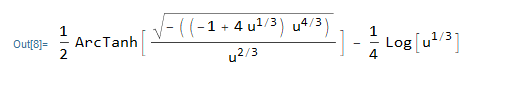It is good that Maple can solve this integral, but why simplify gives error on the result? Is there a workaround?

## How to use Physics in Maple 2021?...

I installed Maple 2021 on windows 10. And wanted to try it to see if the hangs I used to have are fixed now.

First I noticed that Physics package does not come pre-installed with Maple 2021, which is little strange. I would have expected Maple 2021 to come with latest Physics version.

Because when I did Physics:-Version() it says "`The "Physics Updates" package is not installed`"

Then I typed  Physics:-Version(latest) to install it, it gives error

Error, (in Physics:-Version) unable to determine the Physics Updates version, could you please report the problem to support@maplesoft.com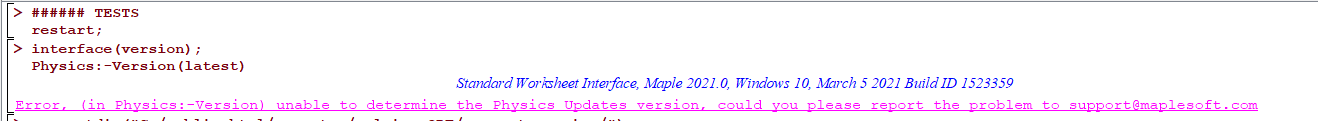I remember something similar in earlier version of Maple but can't find or remember where that post now.

May be Physics needs to be updated at Maple site to work with Maple 2021? I am asking, because Latex() does not work without Physics installed.

Edit:

I think in Maple 2021, latex() now is the same as the earlier Latex() command from Physics? I remember a post saying this now. Since I see now latex() have different help page from old latex() help page.

So may be that is why Latex() did not work. I can easily change this in my code to change it to use latex() instead of Latex() in this case.

Windows 10

﻿